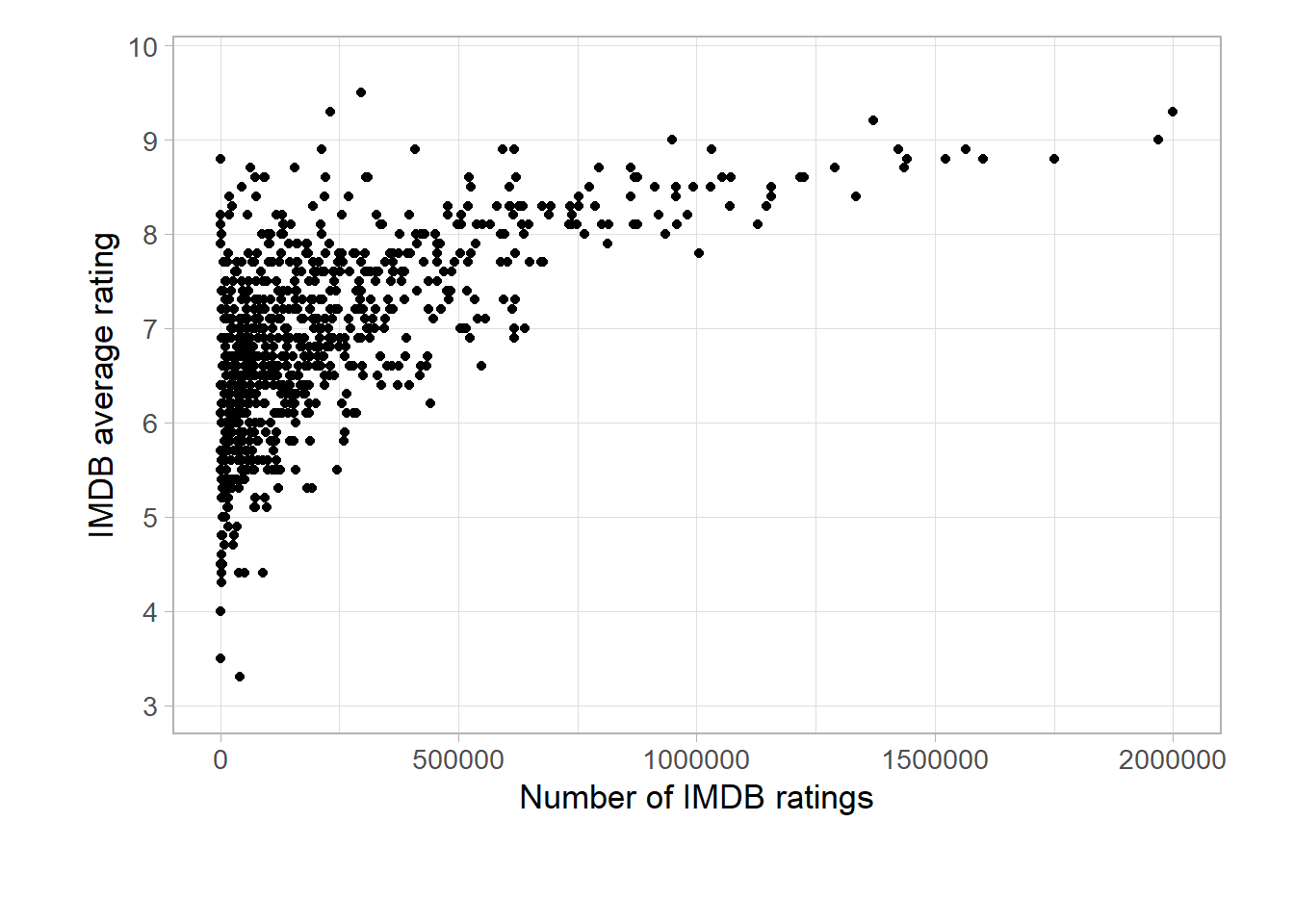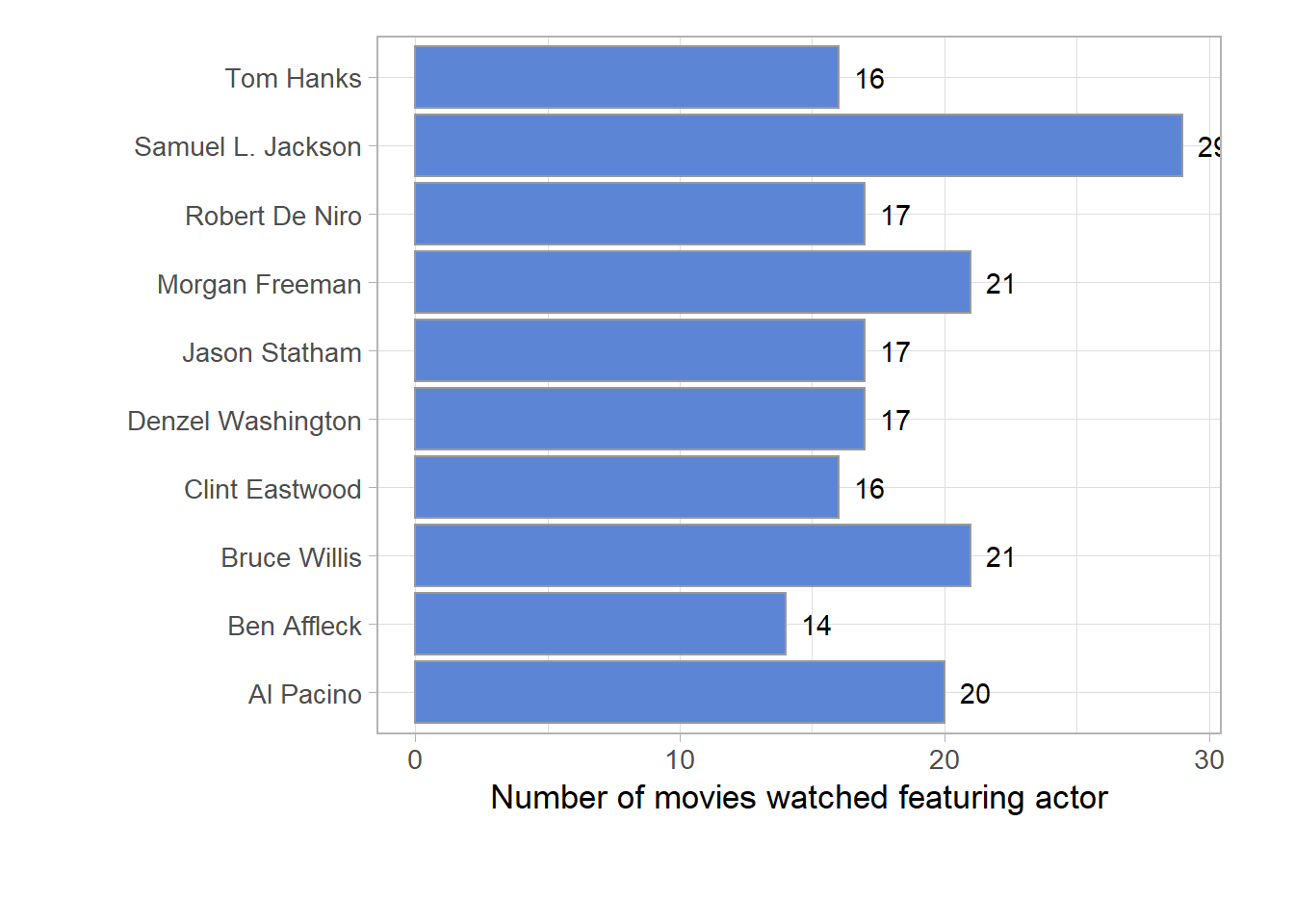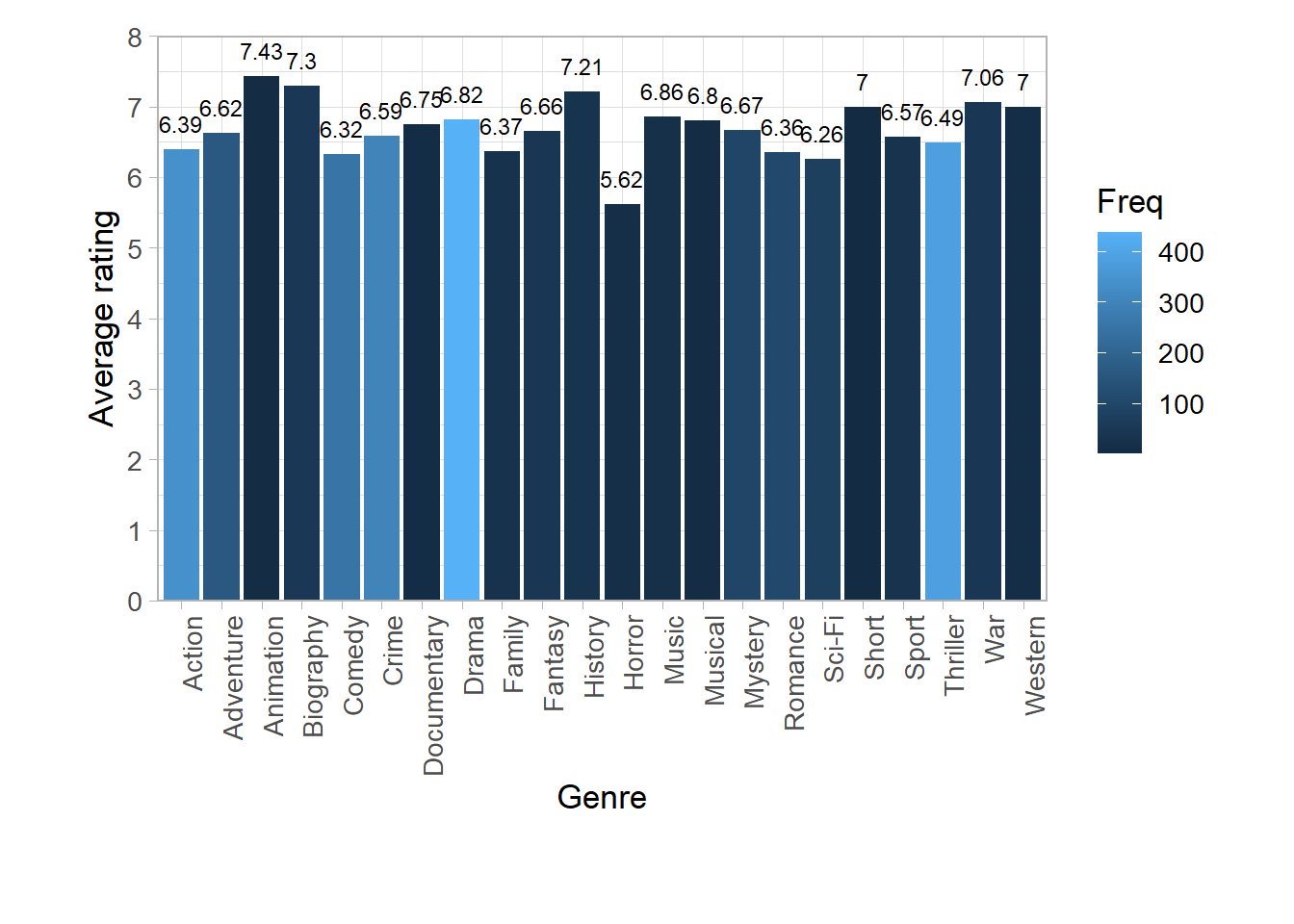# Visualize and analyse IMDB ratings with R (part 2)

This post is part of a series of posts to analyse the digital me.

In my previous post about analysing IMDB ratings in this series I explored some of the data I collected about my movie preferences, also compared to the ratings other IMDB raters.

In this post I will dig a little deeper to learn more about my own personal movie preferences.

## Does popularity of a movie impact my rating?

Some movies are very mainstream, other are more niche or an “acquired” taste. The popularity of a movie can be determined in IMDB by the number of reviews a movie has received. Let’s first see how the number of IMDB reviews relates to the average IMDB rating. We can do that by building a sctaterplot.

``````theme_data <- theme_light(base_size = 13) + theme(plot.margin=unit(c(0.5,1,1.5,1.2),"cm"))
color_bar <- "#999999"
color_fill <- "#5c85d6"
ggplot(data_imdb, aes(x = imdb_ratings, y = imdb_rating)) +
geom_point() +
#scale_x_discrete(limit = c(1930, 1940, 1950, 1960, 1970, 1980, 1990, 2000, 2010)) +
scale_y_discrete(limit = c(3:10)) +
ylab("IMDB average rating") +
xlab("Number of IMDB ratings") +
theme_data``````In this scatterplot you can see that most of the movies are clustered around a low number of reviews and are receiving average ratings between 6 and 7. It’s interesting to see that the movies with most IMDB ratings are all highly rated. Let’s check out which movies these are.

``head(arrange(data_imdb[,c("title", "imdb_ratings", "imdb_rating")],desc(imdb_ratings)),10)``
``````##                                                title imdb_ratings imdb_rating
## 1                           The Shawshank Redemption      2000322         9.3
## 2                                    The Dark Knight      1969523         9.0
## 3                                          Inception      1750193         8.8
## 4                                         Fight Club      1600985         8.8
## 5                                       Pulp Fiction      1565763         8.9
## 6                                       Forrest Gump      1522234         8.8
## 7  The Lord of the Rings: The Fellowship of the Ring      1441273         8.8
## 8                                         The Matrix      1435239         8.7
## 9      The Lord of the Rings: The Return of the King      1424377         8.9
## 10                                     The Godfather      1370383         9.2``````

So Shawshank Redemption and Dark Knight are the most reviewed movies I have rated myself. And they are both highly rated.

### Understanding the probability of number of ratings to impact my rating

The probability can be calculated with a p-value.

``cor(as.numeric(data_imdb\$imdb_ratings), as.numeric(data_imdb\$myRating))``
``##  0.4478298``

As you can see, the p-value is 0.4478298. This means that the number of ratings on IMDB will have a 45% chance of having no effect on my own rating. So there is a pretty high probability that the number of ratings will have an effect on my rating (52%).

## My favorite actors

I always think I know which actors I like and put their movies on the top of my watchlist. Let’s check out if I actually rate the movies my favorite actors with the highest scores too.

For each movies I rate, there are a maximum of 15 actors added to the dataset. I have added them all to one column, pipe separated. I will use the data.table and stringr package to split the data in the cast column and learn more about the actors in the movies I reviewed. I will create a new dataframe with a count of the the actors (unique_actor_count) and find out how many actors are in this dataframe (unique_actors). After that, I will create a new dataframe with my top 10 most watched actors and visualise it in a barchart for easy interpretation.

``````library('data.table')
library('stringr')
as.character(data_imdb\$imdb_cast) -> data_imdb\$imdb_cast
data.frame(table(unlist(strsplit(data_imdb\$imdb_cast, "[|]")))) -> unique_actor_count``````
``````nrow(unique_actor_count) -> unique_actors

ggplot(data = unique_actor_count_top10, aes(x = Var1, y = Freq)) +
geom_bar(stat = "identity", fill=color_fill, color = color_bar)+
coord_flip() +
geom_text(aes(label = Freq), position=position_dodge(width=1), hjust = -0.5) +
theme(axis.text.x = element_text(angle = 90, hjust = 1)) +
ylab("Number of movies watched featuring actor") +
xlab("") +
theme_data``````It turns out more than 8000 actors have made it to my dataframe and the one I have viewed more than others was Samuel L. Jackson. I’m surprised to see only male actors made it to my top 10. Not sure how that happened…

## My favorite movie genres

Just like my favorite actors, I can also learn more about favorite genres. The data is setup in the same way, so for now I will go straight to the visualisations.

``````as.character(data_imdb\$imdb_genres) -> data_imdb\$imdb_genres
data.frame(table(unlist(strsplit(data_imdb\$imdb_genres, "[|]")))) -> unique_genres_count
filter(unique_genres_count, !grepl(paste(genre_dirt, collapse="|"), Var1)) -> unique_genres_count
nrow(unique_genres_count)``````
``##  22``

Just like actors, it is possible for a movie to have multiple genres. I have made them unique and can now see that I only have 22 genres in the dataset.

Let’s see what the average rating is per genre and the number of movies I have watched by genre.

``````head(arrange(unique_genres_count,desc(Freq)),10) -> unique_genres_count_top10

unique_genres_count\$Var1 -> genre_group

df_mean_genre <- data.frame()
for(v in genre_group){
data_imdb %>%
filter(str_detect(imdb_genres, v)) %>%
select(myRating) -> df7
round(mean(df7\$myRating),2) ->mean_genre
df8 <- data.table("genre" = v, "mean" = mean_genre)
df_mean_genre<-rbind(df_mean_genre,df8)
}

genre_overview = merge(unique_genres_count, df_mean_genre, by.x=c("Var1"), by.y=c("genre"))

ggplot(data = genre_overview, aes(x = Var1, y = mean, fill = Freq)) +
geom_bar(stat = "identity")+
geom_text(aes(label = mean), size = 3, position=position_dodge(width=1), vjust = -1) +
scale_y_continuous(expand = c(0, 0),breaks = seq(0, 10, 1), limits = c(0,8)) +
ylab("Average rating") +
xlab("Genre") +
theme_data +
theme(axis.text.x = element_text(angle = 90, hjust = 1))``````I really enjoy a great animation movie (brings out the child in me), but I hardly watch them. Horror is not my preferred genre and that shows in the number of horror movies I have seen and how I rate them.